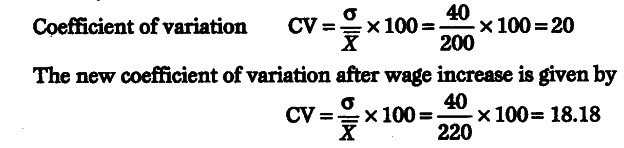# What is the new average daily wage and standard deviation?

Average daily wage of 50 workers of a factory was Rs 200 with a standard deviation of Rs 40. Each worker is given a raise oft 20. What is the new average daily wage and standard deviation? Have the wages become more or less uniform?

Thus, mean increases by the amount of increase in wage of each worker as the absolute increase was equal for all.Standard deviation will remain the same that is ?40 as standard deviation is independent of origin and hence an addition of equal amount in all the values will not cause any change in the standard deviation. ’
Uniformity of wages can he seen by coefficient of variation.
Previously, the coefficient of variation wasThis shows that wages have become more uniform now as the new coefficient of variation is lower.